• 博主最近在看卡尔曼滤波算法，个人认为在卡尔曼滤波算法中最核心的部分莫过于高维高斯联合概率分布的性质，因此打算将这些性质整理成博客记录下来方便自己今后的学习，如果有哪里不对，欢迎各位读者指正。...
博主最近在看卡尔曼滤波算法，个人认为在卡尔曼滤波算法中最核心的部分莫过于高维高斯联合概率分布的性质，因此打算将这些性质整理成博客记录下来方便自己今后的学习，如果有哪里不对，欢迎各位读者指正。
一 引理
​			这里我引入一个定理，这个定理不在本博客证明，因为它很直观，便于理解。
​			假设随机变量$X$服从均值为$\mu$，协方差矩阵为$\Sigma$的高斯分布(为了更具有一般性，这里的均值是一个向量，协方差是一个矩阵)。随机变量$Y=AX+B$(这里的矩阵$A$和$B$都是常值矩阵)，则结论是$Y$也服从于一个高维高斯分布，它的均值是$A\mu+B$，协方差矩阵是$A\Sigma{A^{T}}$。
二 推导
​			设$p$维随机变量$X=(x_1,x_2,\dots,x_p)^{T}$服从均值$\mu=(\mu_1,\mu_2,\dots,\mu_p)^{T}$，协方差矩阵为式(2-1)的高斯分布，现在我们将随机变量$X$切分为两个随机变量，第一个随机变量取随机变量$X$的前$m$维记为$X_a$，对应的均值为$\mu_a$。第二个随机变量取随机变量$X$的后$n$维记为$X_b$，对应的均值为$\mu_b$，且满足($m+n=p$)。则随机变量$X$可以写成$X=(X_a,X_b)^{T}$，均值可以写成$\mu=(\mu_a,\mu_b)^{T}$，协方差矩阵可写成式(2-2)。
$\Sigma= \left\{ \begin{matrix} \sigma_{11} & \sigma_{12} & \dots & \sigma_{1p} \\ \sigma_{21} & \sigma_{22} & \dots & \sigma_{2p} \\ \vdots & \vdots & \dots & \sigma_{3p} \\ \sigma_{p1} & \sigma_{p2} & \dots & \sigma_{pp} \end{matrix} \right\} \tag{2-1}$
$\Sigma= \left\{ \begin{matrix} \Sigma_{aa}&\Sigma_{ab}\\ \Sigma_{ba}&\Sigma_{bb} \end{matrix} \right\} \tag{2-2}$
​			现在的问题是随机变量$X_a$以及在给定$X_a$的条件下$X_b$服从什么样参数的分布?
​			为了使用引入的定理，这里我们构造出$X_a$与$X$之间的关系，即$X_a=(I_m,0_n)X$。由此可以看出，$X_a$可以由$X$线性表出，则$X_a$服从高斯分布，均值和协方差矩阵求解见式(2-3)。
$E[X_a]=(I_m,0)\mu=\mu_a\\ Var[X_a]= \begin{pmatrix} I_m&0 \end{pmatrix} \begin{pmatrix} \Sigma_{aa}&\Sigma_{ab}\\ \Sigma_{ba}&\Sigma_{bb} \end{pmatrix} \begin{pmatrix} I_m^{T}\\ 0\\ \end{pmatrix}= \begin{pmatrix} \Sigma_{aa}&\Sigma_{ab} \end{pmatrix} \begin{pmatrix} I_m\\ 0 \end{pmatrix} =\Sigma_{aa} \tag{2-3}$
所以$X_a$服从于均值为$\mu_a$，协方差为$\Sigma_{aa}$的高斯分布。
现在做一下变量替换，见式(2-4)，这里的替换纯属是为了后面计算方便，读者不必纠结于此。
$X_{b.a}=X_b-\Sigma_{ba}\Sigma_{aa}^{-1}X_{a}\\ \mu_{b.a}=\mu_b-\Sigma_{ba}\Sigma_{aa}^{-1}\mu_a\\ \Sigma_{bb.a}=\Sigma_{ba}-\Sigma_{aa}^{-1}\Sigma_{ab} \tag{2-4}$
于是$X_{b.a}$可以表示为$(-\Sigma_{ba}\Sigma_{aa}^{-1},I_n)X$。并且可以验证，$X_{b.a}$的期望为$\mu_{b.a}$，协方差为$\Sigma_{bb.a}$。因此$X_b=X_{b.a}+\Sigma_{ba}\Sigma_{aa}^{-1}X_a$。所以在给定$X_a$的前提下，$E[X_{b}|X_a]=\mu_{b.a}+\Sigma_{ba}\Sigma_{aa}^{-1}\mu_a$，$Var[X_b|X_a]=Var[X_{b.a}]=\Sigma_{bb.a}$。


展开全文• 一、概率分布 离散型变量的概率分布可以用 概率质量函数(probability mass function, PMF) 来描述。我们通常用大写字母 P 来表示概率质量函数。通常每一个随机变量都会有 一个不同的概率质量函数，并且读者必须根据...
一、概率分布

离散型变量的概率分布可以用 概率质量函数(probability mass function, PMF) 来描述。我们通常用大写字母 P 来表示概率质量函数。通常每一个随机变量都会有 一个不同的概率质量函数，并且读者必须根据随机变量来推断所使用的 PMF，而不 是根据函数的名称来推断;例如，P(x) 通常和 P(y) 不一样。

概率质量函数将随机变量能够取得的每个状态映射到随机变量取得该状态的概 率。x = x 的概率用 P(x) 来表示，概率为 1 表示 x = x 是确定的，概率为 0 表示 x = x 是不可能发生的。有时为了使得PMF的使用不相互混淆，我们会明确写出随 机变量的名称:P (x = x)。有时我们会先定义一个随机变量，然后用 ∼ 符号来说明它遵循的分布:x ∼ P (x)。

概率质量函数可以同时作用于多个随机变量。这种多个变量的概率分布被称为联合概率分布(joint probability distribution)。P (x = x, y = y) 表示 x = x 和 y = y 同时发生的概率。我们也可以简写为 P (x, y)。

当我们研究的对象是连续型随机变量时，我们用 概率密度函数(probability density function, PDF)而不是概率质量函数来描述它的概率分布。概率密度函数 p(x) 并没有直接对特定的状态给出概率，相对的，它给出了落在面积为 δx 的无限小的区域内的概率为：

p(x)δx

我们可以对概率密度函数求积分来获得点集的真实概率质量。特别地，x 落在 集合 S 中的概率可以通过 p(x) 对这个集合求积分来得到。在单变量的例子中，x 落在区间 [a, b] 的概率是：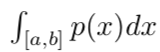为了给出一个连续型随机变量的 PDF 的例子，我们可以考虑实数区间上的均匀分布。我们可以使用函数 u(x; a, b)，其中 a 和 b 是区间的端点且满足 b > a。符号“;’’ 表示 ‘‘以什么为参数’’.我们把 x 作为函数的自变量，a 和 b 作为定义函数的参数。为了确保区间外没有概率，我们对所有的 x ∉ [a, b]，令 u(x; a, b) = 0。在 [a, b]内，有: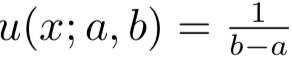我们可以看出任何一点都非负。另外，它的积分为 1。我们 b−a通常用 x ∼ U(a,b) 表示 x 在 [a,b] 上是均匀分布的。

二、边缘概率

有时候，我们知道了一组变量的联合概率分布，但想要了解其中一个子集的概率分布。这种定义在子集上的概率分布被称为 边缘概率分布(marginal probability distribution)。

例如，假设有离散型随机变量 x 和 y，并且我们知道 P(x,y)。我们可以依据下 面的 求和法则(sum rule)来计算 P (x):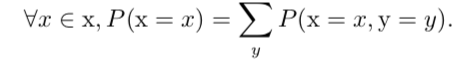‘‘边缘概率’’ 的名称来源于手算边缘概率的计算过程。当 P(x,y) 的每个值被写 在由每行表示不同的 x 值，每列表示不同的 y 值形成的网格中时，对网格中的每行 求和是很自然的事情，然后将求和的结果 P(x) 写在每行右边的纸的边缘处。

对于连续型变量，我们需要用积分替代求和: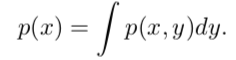三、条件概率

在很多情况下，我们感兴趣的是某个事件在给定其他事件发生时出现的概率。这种概率叫做条件概率。我们将给定 x = x，y = y 发生的条件概率记为 P (y = y | x = x)。这个条件概率可以通过下面的公式计算: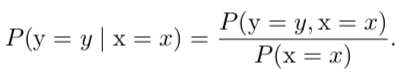条件概率只在 P (x = x) > 0 时有定义。我们不能计算给定在永远不会发生的事件上的条件概率。

这里需要注意的是，不要把条件概率和计算当采用某个动作后会发生什么相混淆。假定某个人说德语，那么他是德国人的条件概率是非常高的，但是如果随机选择的一个人会说德语，他的国籍不会因此而改变。

条件概率的链式法则：

任何多维随机变量的联合概率分布，都可以分解成只有一个变量的条件概率相乘的形式: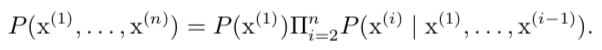例如：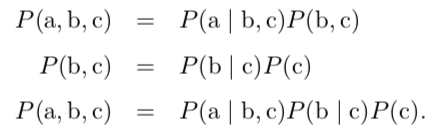四、期望、方差、协方差

函数 f(x) 关于某分布 P(x) 的 期望(expectation)或者 期望值(expected value)是指，当 x 由 P 产生，f 作用于 x 时，f(x) 的平均值。对于离散型随机变量，这可以通过求和得到: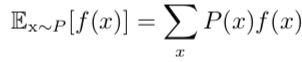连续型通过积分得到：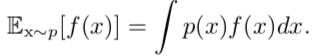当概率分布在上下文中指明时，我们可以只写出期望作用的随机变量的名称来进行简化，例如 Ex[f(x)]。如果期望作用的随机变量也很明确，我们可以完全不写脚标， 就像 E[f(x)]。默认地，我们假设 E[·] 表示对方括号内的所有随机变量的值求平均。 类似的，当没有歧义时，我们还可以省略方括号。

期望是线性的，例如：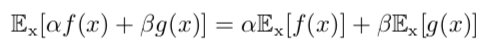方差(variance)衡量的是当我们对 x 依据它的概率分布进行采样时，随机变 量 x 的函数值会呈现多大的差异: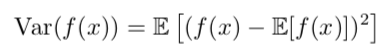当方差很小时，f(x) 的值形成的簇比较接近它们的期望值。

标准差是方差的算术平方根。

协方差(covariance)在某种意义上给出了两个变量线性相关性的强度以及这些变量的尺度: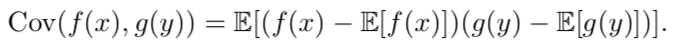协方差的绝对值如果很大则意味着变量值变化很大并且它们同时距离各自的均值很远。如果协方差是正的，那么两个变量都倾向于同时取得相对较大的值。如果协方差是负的，那么其中一个变量倾向于取得相对较大的值的同时，另一个变量倾向于 取得相对较小的值，反之亦然。其他的衡量指标如相关系数(correlation)将每个变量的贡献归一化，为了只衡量变量的相关性而不受各个变量尺度大小的影响。

协方差和相关性是有联系的，但实际上是不同的概念。它们是有联系的，因为两个变量如果相互独立那么它们的协方差为零，如果两个变量的协方差不为零那么它们一定是相关的。然而，独立性又是和协方差完全不同的性质。两个变量如果协方差为零，它们之间一定没有线性关系。独立性比零协方差的要求更强，因为独立性还排除了非线性的关系。两个变量相互依赖但具有零协方差是可能的。

随机向量 x ∈  的 协方差矩阵(covariance matrix)是一个 n × n 的矩阵，并且满足：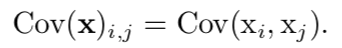协方差矩阵的对角元是方差: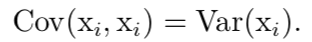展开全文条件概率
• ## 机器学习-联合概率分布笔记

万次阅读 多人点赞 2017-10-24 18:13:56
1.什么是联合概率分布？  联合概率分布简称联合分布，是两个及以上随机变量组成的随机向量的概率分布。根据随机变量的不同，联合概率分布的表示形式也不同。对于离散型随机变量，联合概率分布可以以列表的形式表示...
1.什么是联合概率分布？
联合概率分布简称联合分布，是两个及以上随机变量组成的随机向量的概率分布。根据随机变量的不同，联合概率分布的表示形式也不同。对于离散型随机变量，联合概率分布可以以列表的形式表示，也可以以函数的形式表示；对于连续型随机变量，联合概率分布通过一非负函数的积分表示。

2. 举例说明联合概率分布
打靶时命中的坐标（x，y）的概率分布就是联合概率分布（涉及两个随机变量），其他同样类比

3.离散型联合概率分布
以下摘自百度百科：

对于二维离散随机向量，设X和Y都是离散型随机变量，和分别是X和Y的一切可能的几何，则X和Y的联合概率分布可以表示为如下图的列联表，也可以表示为如下的函数形式其中变量X和Y的联合分布完全决定X的概率分布和Y的概率分布（称作联合分布的边缘分布）：对于多维（维数大于等于3）离散型随机变量的联合概率分布以此类推。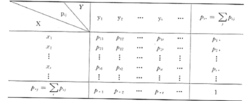题目详解：

假设X和Y都是离散型分布

先看X的概率分布：
X      0        1
p     0.4     0.6

再看Y的概率分布：
Y     0          1      2
p    0.25    0.5    0.25

又因为X与Y相互独立，所以（X,Y）的联合概率分布为：
X\Y      0         1          2
0       0.1      0.2       0.1
1      0.15     0.3      0.15

P(X＜Y)=P(X=0，Y=1)+P(X=0，Y=2)+P(X=1，Y=2)=0.2+0.1+0.15=0.45

4.连续型联合概率分布

对于二维连续随机向量，设X和Y为连续型随机变量，其联合概率分布，或连续型随机变量的概率分布通过一非负函数的积分表示，称函数为联合概率密度函数。
两者的关系如下：不但完全决定X和Y的联合概率分布，而且完全决定X的概率分布和Y的概率分布，以和分别表示X和Y的概率密度，则对于多维（维数大于等于3）连续型随机变量的联合概率分布以此类推。


展开全文• 概率和信息论。概率论，表示不确定性声明数学框架。提供量化不确定性方法，提供导出新不确定...信息论量化概率分布不确定性总量。Jaynes(2003)。 机器学习经常处理不确定量，有时处理随机(非确定性)量。20世纪80年代，
概率和信息论。

概率论，表示不确定性声明数学框架。提供量化不确定性方法，提供导出新不确定性声明(statement)公理。人工智能领域，概率法则，AI系统推理，设计算法计算概率论导出表达式。概率和统计理论分析AI系统行为。概率论提出不确定声明，在不确定性存在情况下推理。信息论量化概率分布不确定性总量。Jaynes(2003)。
机器学习经常处理不确定量，有时处理随机(非确定性)量。20世纪80年代，研究人员对概率论量化不确定性提出信服论据。Pearl(1998)。

不确定性来源。被建模系统内存的随机性。不完全观测，确定系统不能观测到所有驱动系统行为变量，也呈随机性。不完全建模，模型舍弃观测信息，导致预测不确定性。简单而不确定规则比复杂而确定规则更实用，即使真正规则是确定的并且建模型系统足够精确容纳复杂规则。

概率论分析事件发生频率。事件可以重复。结果发生概率p，反复无限次，有p比例会导致某个结果。概率表示信任度(degree of belief)。直接与事件发生的频率相联系，频率派概率(frequentist probability)。涉及到确定性水平，贝叶斯概率(Bayesian probability)。不确定性常识推理，列出若干条期望性质，满足唯一方法是贝叶斯概率和频率概率等同。Ramsey(1926)。概率，处理不确定性逻辑扩展。逻辑提供形式化规则，给定命题真假，判断另一些命题真假。概率论提供形式化规则，给定命题似然，计算其他命题为真似然。

随机变量(random variable)。

随机取不同值变量。无格式字体(plain typeface)小写字母表示随机变量，手写体小写字母表示随机变量取值。随机变量对可能状态描述。伴随概率分布批定每个状态可能性。随机变量可以离散或连续。离散随机变量有限或可数无限多状态。可能没有数值。连续随机变量伴随实数值。

概率分布(probability distribution)。

随机变量或一簇随机变量每个状态可能性大小。描述概率分布方式取决随机变量离散还是连续。

离散型变量和概率质量函数。离散弄变量概率分布用概率质量函数(probability mass function,PMF)描述。大写字母P表示概率质量函数。每个随机变量有一个不同概率质量函数，根据随机变量推断所用PMF。概率质量函数将随机变量每个状态映射到随机变量取该状态概率。x=x概率用P(x)表示，概率1表示x=x确定，概率0表示x=x不可能发生。明确写出随机变量名称，P(x=x)。定义随机变量，用~符号说明遵循分布，x~P(x)。概率质量同时作用多个随机变量。多个变量概率分布为联合概率分布(joint probability distribution)。P(x=x,y=y)表示x=x和y=y同时发生概率。简写P(x,y)。函数P是随机变量x的PMF，P定义域必须是x所有可能状态集合。FORALL(x) ELEMENT(X)，0<=P(x)<=1。不可能发生事件概率为0，不存在概率更低状态。确保一定发生事件概率为1,不存在概率更高状态。SUM(x  ELEMENT(X),P(x))=1。归一化(normalized)。

离散型随机变量x有k个不同状态，x均匀分布(uniform distribution)，每个状态均等可能。PMF，P(x=x i)=1/k。所有i成立。k是一个正整数，1/k是正的。SUM(i, P(x=x i))=SUM(i, 1/k)=k/k=1。分布满足归一化条件。
连续型变量和概率密度函数。连续型随机变量，概率密度函数(probability density function,PDF)描述概率分布。函数p是概率密度函数。p定义域是x所有可能状态集合。FORALL(x) ELEMENT(X)，P(x)>=0，不要求p(x)<=1。INTEGRAL(p(x)dx)=1 。概率密度函数p(x)给出落在面积为DELTA(x)无限小区域内概率为p(x)DELTA(x)。概率密度函数求积分，获得点集真实概率质量。x落在集合S中的概率，p(x)对集合求积分得到。单变量，x落在区间[a,b]概率是INTEGRAL([a,b],p(x)dx) 。

实数区间均匀分布。函数u(x;a,b)，a和b 是区间端点，满足b>a。符号”;”表示以什么为参数。x作函数自变量，a和b作定义函数参数。确保区间外没有概率，所有x NOTELEMENT([a,b])，令u(x;a,b)=0。在[a,b]内，u(x;a,b)=1/(b-a)。任何一点都非负。积分为1。x~U(a,b)表示x在[a,b]上均匀分布。

边缘概率。

定义在子集上的概率分布为边缘概率分布(marginal probability distribution)。离散型随机变量x和y，知道P(x,y)，求和法则(sum rule)计算FORALL(x) ELEMENT(X),P(x=x)=SUM(y,P(x=x,y=y)) 。边缘概率名称来源手算边缘概率计算过程。P(x,y)每个值被写在每行表示不同x值、每列表示不同y值网格中，对网络中每行求和，求和结果P(x)写在每行右边纸边缘处。连续型变量，用积分替代求和，p(x)=INTEGRAL(p(x,y)dy。

条件概率。

某个事件上在给定其他事件发生时出现概率。给定x=x,y=y发生条件概率记P(y=y|x=x)。P(y=y|x=x)=P(y=y,x=x)/P(x=x)。条件概率只在P(x=x)>0有定义。不能计算给定在永远不会发生事件上上的条件概率。不要把条件概率和计算当采用某个动作后会发生什么相混淆。

条件概率链式法则。

任何多维随机变量联合概率分布，都可以分解成只有一个变量的条件概率相乘形式。P(x (1) ,…,x (n) )=P(x (1) )PRODUCT(i=2,n,P(x (i) |x (i) ,…,x (i-1) ))。概率链式法则(chain rule)或乘法法则(product rule)。从条件概率定义得到，使用两次定义得到，P(a,b,c)=P(a|b,c)P(b,c)。P(b,c)=P(b|c)P(c)。P(a,b,c)=P(a|b,c)P(b|c)P(c)。

独立性和条件独立性。

两个随机变量x和y，概率分布表示成两个因子乘积形式，一个因子只包含x，另一个因子只包含y，两个随机变量相互独立(independent)。FORALL(x) ELEMENT(x),y ELEMENT(y),z ELEMENT(z),p(x=x,y=y)=p(x=x)p(y=y)。x和y的条件概率分布对于z的每一个值都写成乘积形式，随机变量x和y在给定随机变量z时条件独立(conditionally independent)。FORALL(x) ELEMENT(x),y ELEMENT(y),z ELEMENT(z),p(x=x,y=y|z=z)=p(x=x|z=z)p(y=y|z=z)。简化形式表示独立笥和条件独立性，x UPTACK(y)表示x和y相互独立，x UPTACK(y)|z表示x和y在给定z时条件独立。

期望、方差和协方差。

函数f(x)关于某分布P(x)的期望(expectation)或期望值(expected value)，当x由P产生，f作用于x，f(x)的平均值。对于离散型随机变量，求和得到，E x~P [f(x)]=SUM(x,P(x)f(x))。连续型随机变量，求积分得到，E x~p [f(x)]=INTEGRAL(p(x)f(x)dx) 。概率分布在上下文指明，只写出期望作用随机变量名称简化，Ex[f(x)]。期望作用随机变量明确，不写脚标，E[f(x)]。默认，假设E[.]表示对方括号内所有随机变量值求平均。没有歧义时，可以省略方括号。期望线性，E x [af(x)+bg(x)]=aEx[f(x)]+bE x [g(x)]。a和b不依赖x。

方差(variance)衡量，x依据概率分布采样时，随机变量x函数值差异。Var(f(x))=E[(f(x)-E[f(x)]) 2 ]。方差很小时，f(x)值形成簇比较接近期望值。方差的平方根为标准差(standard deviation)。

协方差(covariance)，给出两个变量线性相关性强度及变量尺度。Cov(f(x),g(y))=E[(f(x)-E[f(x)])(g(y)-E[g(y)])]。协方差绝对值很大，变量值变化很大，距离各自的均值很远。协方差为正，两个变量倾向于同时取得相对较大值。协方差为负，一个变量倾向于取较大值，另一个变量倾向于取较小值。其他衡量指标，相关系数(correlation)，每个变量贡献归一化，只衡量变量相关性，不受各个变量尺度大小影响。

协方差和相关性有联系，是不同概念。联系。两个变量互相独立，协方差为零。两个变量协义差不为零，一定相关。独立性和协方差性质完全不同。两个变量协方差为零，一定没有相互依赖，但具有零协方差可能。从区间[-1,1]均匀分布采样一个实数x，对一个随机变量s采样。s以1/2概率值为1,否则为-1。令y-sx生成一个随机变量y。x和y不相互独立，x完全决定y尺度.Cov(x,y)=0。

随机向量x ELEMENT(R n )协方差矩阵(convariance matrix)是n*n矩阵，满足，Cov(x) i,j =Cov(x i ,x j )。协方差矩阵对角元是方差，Cov(x i ,x i )=Var(x i )。

参考资料：

《深度学习》

欢迎推荐上海机器学习工作机会，我的微信：qingxingfengzi

我有一个微信群，欢迎一起学深度学习。
展开全文机器学习 深度学习
• 举个例子，丢两枚硬币，正面为1，反面为0 ...联合概率：P1（1，0），P2（0，1），P3（1，1），P4（0，0），分别代表4个联合概率 边缘概率：P1+P3表示不管第二个硬币的值，第一个硬币是正面的概率，是一种边缘概率 ...
• 概率和信息论。 概率论，表示不确定性声明数学框架。提供量化不确定性方法，提供导出新不确定性声明(statement)公理。人工智能领域，概率法则，AI系统推理，设计算法计算概率论导出表达式。概率和统计理论分析AI...
• 相信你对变量这个概念并不陌生，数学方程式编程代码里经常会用到变量。那什么是变量呢？我们在概率中常说的随机变量（ random variable）普通的变量（variable）又有什么...
• ## 最大熵和概率分布

千次阅读 2019-06-02 13:04:55
概率论 我们需要描述一组数据时候，本质上需要描述每一个点。但是如果我们可以用分布去表示...p(k)=pk(1−p)(1−k),k∈{0,1}p(k)=p^k(1-p)^{(1-k)}, k\in\{0, 1\}p(k)=pk(1−p)(1−k),k∈{0,1} ，期望：ppp，方差：p...机器学习
• 最近遇到了一个联合对数正态分布的相关系数的问题，搜遍全网无果，索性自己动手。本文借鉴了这个知乎回答 首先我们有二维正态分布： X,Y∼BVN(μx,μy,σx2,σy2,ρxy)X,Y\sim \mathbf{BVN}(\mu_x,\mu_y,\sigma_x^2,...数学
• 文章目录1 基本概念2 常用概率分布2.1 伯努利分布2.2 贝塔分布2.3 分类分布2.4 狄利克雷分布2.5 一元正态分布2.6 正态逆伽马分布2.7 多元正态分布2.8 正态逆维希特分布2.9 ...  2）联合概率：假设两个随机变量xxxyy机器学习
• ## 离散型概率分布

千次阅读 2019-03-21 16:24:16
1.2 离散型概率分布 1.3 数学期望与方差、标准差 1.3.1 数学期望 1.3.2 方差 1.3.3 标准差 1.3.4 线性变换的通用公式 1.3.5 独立观测值 1.4 二项概率分布（binomial probability distribution） 1.5 泊松...数据分析
• 本章主要介绍各种概率分布，包括伯努利分布、Beta分布、狄利克雷分布、高斯分布（重点）、指数族分布和非参数化方法估计的概率分布等读书笔记 KL散度
• 11.正态分布之后专开一篇写正态分布的各种知识点。这里仅说下R里面用正态分布函数的一个注意点，一般书面写正态分布为 ，如 ，其中 ，而R语言中写为 ，即后面的参数写标准差，而非方差。基本密度函数期望方差特征...
• 如果两个变量（属性/特征）独立，则联合概率是两个变量边缘概率的乘积，即 。则在X的条件下Y独立，根据product rule，得到条件概率   。若两个盒子的两种水果占比一样，则 , 则苹果与选择的盒子无关。 2、贝叶斯...
• ## 概率分布之二项分布与多项分布

万次阅读 多人点赞 2017-02-10 22:38:02
1. 伯努利分布(Bernoulli distribution)  伯努利分布又称二点分布或0-1分布，即一次试验只有正例反例两种可能，以随机变量表示就是X只能取0或1，伯努利试验是只有两种可能结果的单次随机试验
• 标签： 机器学习概率分布这章主要介绍一些常用的分布模型。 对于变量x的N次观测形成独立同分布的向量x⃗ =(x1,...,xN)\vec x=(x_1,...,x_N)，以此对x做一个密度估计，出p(x)p(x). 密度估计⎧⎩⎨⎪⎪⎪⎪参数...模式识别 机器学习
• 概率,期望，方差 　只有一个变量时 　F(x<=a) =∫-∞af(x)dx 　当区间取负无穷到正无穷时积分为1 　推广到多元之后: 　 　同理，当区间取满整个空间时，积分为1 　f被称为概率密度函数 　边缘分布函数...
• 计算机视觉是人工智能的重要分支，也是一门具有很强综合性的学科，涉及计算机科学与工程、信号处理、光学、应用数学、统计学、神经生理学认知科学等学科。 OpenCV 是一个开源、跨平台的计算机视觉库，基于 C/C++...计算机视觉 全概率公式 贝叶斯定理
• 随机变量及其概率分布 独立性 期望方差协方差 常用概率分布 常用函数 贝叶斯规则 信息论基础 结构化概率模型 如果这篇文章对你有一点小小的帮助，请给个关注喔~我会非常开心的~ 随机变量及其概率分布 ...机器学习 随机变量
• 概率论相对来说简单一点，经常是计算随机事件的概率或者二维随机变量，还有数学期望，大部分看清楚题目条件后直接套公式就行： 协方差的公式也要牢记： ③一般考点（分值低，要么太难，要么太偏） 练习题：历年真题...
• 对非经典分布的随机变量，当然可以按博文《自定义离散型分布》中介绍的方法，自定义rv_discrete（离散型）或rv_continuos（连续型）的子类（详见博文《自定义连续型分布》），然后调用其expect函数计算数学期望。...
• ## 联合概率密度函数

千次阅读 2020-09-25 15:24:46
条件概率密度函数： 边缘概率密度函数： 随机变量G的条件期望
• 这章主要介绍概率分布及其特性。这些基本的分布可以像堆积木一样形成更复杂的模型。...首先介绍离散型贝努力分布、二项式分布和多项式分布和连续型的Gaussian分布。一个特殊 的问题就是参数化估计问题，根...
• 第二章主要介绍几个重要的概率分布及其特性。 1. 二值变量的概率分布  假设一个二元随机变量，用参数表示的概率为：。 （1）伯努利分布（Bernoulli distribution）  概率分布函数： ...
• 转自：... 1. 二值变量的概率分布  假设一个二元随机变量，用参数表示的概率为：。 （1）伯努利分布（Bernoulli distribution）  概率分布函数：  期望：  方差：
• 本博客为（系列二）的笔记，对应的视频是...、【(系列二) 数学基础-概率-高斯分布4-局限性】、【(系列二) 数学基础-概率-高斯分布5-求边缘概率以及条件概率】、【(系列二) 数学基础-概率-高斯分布6-求联合概率分布】。机器学习
• 0前言 最近在温习统计学习方法，比较针对自然语言处理，所以从最大熵开始看，最大熵是后面EM、HMM、CRF的基础...变量分布未知的情况下，同时满足变量所有限制条件，这时变量视为服从均匀分布。 就是书上的例子：......